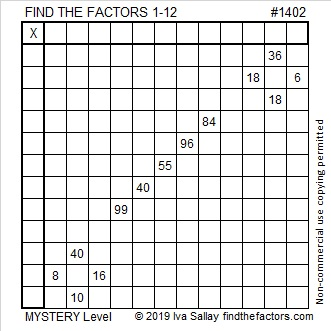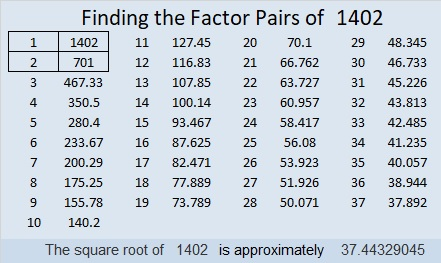# 1402 Mystery Level

Mystery level puzzles may be very difficult or relatively easy. How much trouble will this one be? You’ll have to try it to see!Print the puzzles or type the solution in this excel file: 12 Factors 1389-1403

Now I’ll tell you a little bit about the number 1402:

• 1402 is a composite number.
• Prime factorization: 1402 = 2 × 701.
• 1402 has no exponents greater than 1 in its prime factorization, so √1402 cannot be simplified.
• The exponents in the prime factorization are 1, and 1. Adding one to each exponent and multiplying we get (1 + 1)(1 + 1) = 2 × 2 = 4. Therefore 1402 has exactly 4 factors.
• The factors of 1402 are outlined with their factor pair partners in the graphic below.1402 is the hypotenuse of a Pythagorean triple:
31² + 21² = 1402

1402 is the hypotenuse of a Pythagorean triple:
520-1302-1402 which is 2 times (260-651-701)
and can also be calculated from 2(31)(21), 31² – 21², 31² + 21²

This site uses Akismet to reduce spam. Learn how your comment data is processed.